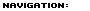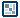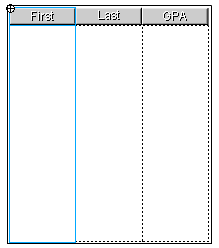FlashComponents Galleries Slideshows Menus Design & Effects Audio & Video User Interface TemplatesIntroduction to XML in Flash
by senocular

Example: Sorted Grades List
Editing XML can be a pain. If you have data that needs to be sorted, you wouldn't want to do that directly from the XML instance. Instead, you could be better off handling it through an array as arrays have sort functions built right in. Because of how a childNodes array works, as an array copy representative of the XML node structure, we can keep XML node references in an array from a childNodes call and just use that to sort and handle our data. If things go horribly wrong, the original data in the XML instance will remain in its original order without disruption. Using a childNodes array still means that we'd be working from XML nodes from the XML instance, but the original order will remain unchanged for the XML instance. This example shows sorting performed on a childNodes array copy that has no effect on the internal XML instance order.

So, starting with this list of students in no particular order:

<?xml version="1.0" ?>
<student first="James" last="Johnson" gpa="3.3" />
<student first="John" last="Smith" gpa="4.0" />
<student first="Serena" last="Williams" gpa="3.8" />
<student first="Indiana" last="Jones" gpa="3.2" />
<student first="Linda" last="Brown" gpa="3.9" />
<student first="Robert" last="Davis" gpa="3.2" />
<student first="Seno" last="Cular" gpa="4.0" />
<student first="Mike" last="Wilson" gpa="3.0" />
<student first="Mary" last="Moore" gpa="3.0" />
<student first="Will" last="Taylor" gpa="2.5" />
<student first="Thomas" last="Anderson" gpa="2.1" />
<student first="David" last="Thomas" gpa="3.7" />

(Which can be found here: grades_1994.xml)

You can use the power of Array.sort() on a childNodes array to rearrange them and display them at will:

[ sort student grades using column headings ]

Preparing the Flash File
The XML document containing students to be sorted uses 3 attributes: first names, last names and gpa. Without using any fancy-schmancy components, we can handle this using three separate, multi-lined (non-wrapping) text fields, one for each attribute. Above each will go the section headings which will also pose as buttons for sorting. Again, one for each attribute labeled accordingly.[ three columns with text fields and a buttons ]

In addition to those buttons, an extra is added to restore order back to that which was specified in the original XML document.[ restore button ]

This is just a copy of all the others with a different name so its really no big deal. And, in fact, the script that is associated with this is far less complicated as well.

ActionScript
The bulk of this example revolves around array sorting. Like many of the simpler examples from before, the XML itself is loaded once and for the most part discarded. Everything after the content is loaded revolves around an array that is assigned as a childNodes array of one of the elements of the XML, more specifically the document root or the grades element.

So lets check out some ActionScript. First, the XML instance:

var students_array;
var grades_xml = new XML();
if (success) {
var grades = this.firstChild;
PopulateLists(students_array);
} else {
}
}

For the most part typical. The difference here is the addition of a students_array variable and the fact that the function being called from the onLoad to handle the loaded content doesn't specifically pass the XML instance or one of its nodes. Rather, it gets the students_array which had been just assigned to a childNodes array from the grade element (which is the XML instance's first child or the XML document's root). This array initially has the order of the XML but can be altered without altering the XML order. The contents of the array are references to XML nodes. If they were altered, as references, they would actually affect the XML.

Here is the PopulateLists function:

PopulateLists = function(xml_array){
first_txt.text = last_txt.text = gpa_txt.text = "";
for (var i=0; i<xml_array.length; i++){
var student = xml_array[i].attributes;
first_txt.text += student.first + "\n";
last_txt.text += student.last + "\n";
gpa_txt.text += student.gpa + "\n";
}
}

First the text in the text fields that make up the columns is cleared by setting them each to "".

first_txt.text = last_txt.text = gpa_txt.text = "";

Then, a for loop is created to cycle through all the elements within the passed array. Since these elements are references to nodes, they will give us access to the XML. Conveniently, a properly named variable is immediately created to reflect that XML information (it could be used to represent the element but since we're dealing with attributes only here, it can be assigned to reference the attributes object).

for (var i=0; i<xml_array.length; i++){
var student = xml_array[i].attributes;
// ...
}

Now all that remains is adding the information from each node within the loop to their respective columns.

first_txt.text += student.first + "\n";
last_txt.text += student.last + "\n";
gpa_txt.text += student.gpa + "\n";

Because each text field is getting a new line of text at the same time, the information will match horizontally. Once the loop is complete, the columns will be populated with all the students in the XML; first and last names and gpa.

All that remains is being able to sort these guys. That poses no considerate problem considering that an array is used to maintain order. However, what that doesn't mean there is no problem. There is still the issue of what to sort by. Considering we have three different categories to sort by, first, last and gpa, it means that every array element within students_array would have to be able to be sorted by any one of those 3 properties. To handle this, we need to create custom sort functions to use with Array.sort(). Otherwise, sort will just sort the XML nodes alphabetically based on their string representations. Custom sort functions would allow us to specifically pin point the part of the node we wish to sort by. Here's what we get in terms of sort functions:

FIRST_SORT = function(a,b){
return a.attributes.first > b.attributes.first;
}
LAST_SORT = function(a,b){
return a.attributes.last > b.attributes.last;
}
GPA_SORT = function(a,b){
return parseFloat(a.attributes.gpa) < parseFloat(b.attributes.gpa);
}

Each is designed to specifically pin point a particular property of the XML nodes from which to base the array sort from. Now we can sort the students_array and pass it in to the PopulateLists function to create a sorted version of the student listing. To handle that we can create the following function:

var current_sort;
SortListBy = function(sortType){
if (current_sort == sortType){
students_array.reverse();
}else{
students_array.sort(sortType);
}
current_sort = sortType;
PopulateLists(students_array);
}

SortListBy takes a sortType, which is actually a sort function, and sorts students_array based on that. You can see a variable current_sort being used to keep track of which sort was used last. This will allow us to reverse the listing if a user attempts to use the same sort more than one time in a row. Following the sorting (or reversing) PopulateLists is called to update the text fields with the newly sorted array.

With that defined, simply add button actions to the column heading buttons specifying the correct sort type for each and your set.

first_btn.onRelease = function(){
SortListBy(FIRST_SORT);
}
last_btn.onRelease = function(){
SortListBy(LAST_SORT);
}
gpa_btn.onRelease = function(){
SortListBy(GPA_SORT);
}

In an effort show that the XML has not been altered, the restore button was added to reset the students_array back to the grade element's current childNodes array. When PopulateLists is called with the new definition of students_array, you can see the sorting has returned to its original unsorted order.

restore_btn.onRelease = function(){